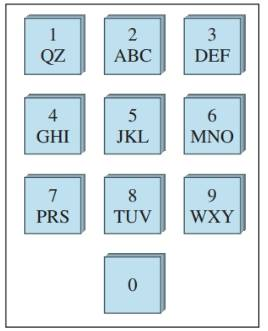Chapter 9.2, Problem 18ES### Discrete Mathematics With Applicat...

5th Edition
EPP + 1 other
ISBN: 9781337694193

#### Solutions

Chapter
Section### Discrete Mathematics With Applicat...

5th Edition
EPP + 1 other
ISBN: 9781337694193
Textbook Problem
11 views

# The following diagram shows the keypad for an automatic teller machine. As you can see, the same sequence of keys represents a variety of different PINs. For instance. 2133, AZDE. and BQ3F are all keyed in exactly the same way.a. How many different PINs are represented by the same sequence of keys as 2133? b. How many different PINs are represented by the same sequence of keys as 5031? c. How many different numeric sequences on the machine contain no repeated digit?

To determine

(a)

The number of different PINs represented by the same sequence of keys as 2133.

Explanation

Given information:

From the keypad shown, we understand that the button containing 2 also contains the letters A,B and C.

Concept used:

The number of different pins, which represent the same sequence of keys as 2133, can be found by selecting any one from the key containing 2,A,B and C. This can be done in 4 ways.

Calculation:

Similarly, the key containing 1 also contains Q and Z, so we can select from the 1,Q and Z in 3 ways

To determine

(b)

The number of different PINs are represented b the same sequence of keys as 5031.

To determine

(c)

The number of numeric sequences that contain no repreated digit.

### Still sussing out bartleby?

Check out a sample textbook solution.

See a sample solution

#### The Solution to Your Study Problems

Bartleby provides explanations to thousands of textbook problems written by our experts, many with advanced degrees!

Get Started